NCERT Solutions (Ex - 13.1) - Direct and Inverse Proportions

# NCERT Solutions (Ex - 13.1) - Direct and Inverse Proportions Notes - Class 8

## Document Description: NCERT Solutions (Ex - 13.1) - Direct and Inverse Proportions for Class 8 2022 is part of Class 8 preparation. The notes and questions for NCERT Solutions (Ex - 13.1) - Direct and Inverse Proportions have been prepared according to the Class 8 exam syllabus. Information about NCERT Solutions (Ex - 13.1) - Direct and Inverse Proportions covers topics like and NCERT Solutions (Ex - 13.1) - Direct and Inverse Proportions Example, for Class 8 2022 Exam. Find important definitions, questions, notes, meanings, examples, exercises and tests below for NCERT Solutions (Ex - 13.1) - Direct and Inverse Proportions.

Introduction of NCERT Solutions (Ex - 13.1) - Direct and Inverse Proportions in English is available as part of our Class 8 preparation & NCERT Solutions (Ex - 13.1) - Direct and Inverse Proportions in Hindi for Class 8 courses. Download more important topics, notes, lectures and mock test series for Class 8 Exam by signing up for free. Class 8: NCERT Solutions (Ex - 13.1) - Direct and Inverse Proportions Notes - Class 8
 1 Crore+ students have signed up on EduRev. Have you?

Exercise 13.1

Question 1:

Following are the car parking charges near a railway station up to: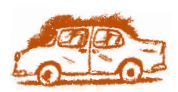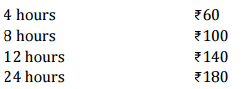Check if the parking charges are in direct proportion to the parking time.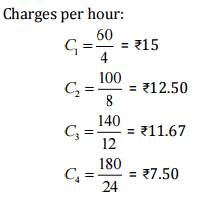Here, the charges per hour are not same, i.e., C1 ≠ C≠ C3 ≠C4

Therefore, the parking charges are not in direct proportion to the parking time.

Question 2:

A mixture of paint is prepared by mixing 1 part of red pigments with 8 parts of base. In the following table, find the parts of base that need to be added.

 Parts of red pigment 1 4 7 12 20 Parts of base 8 — — — —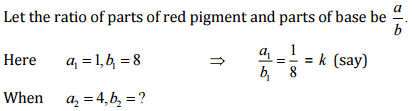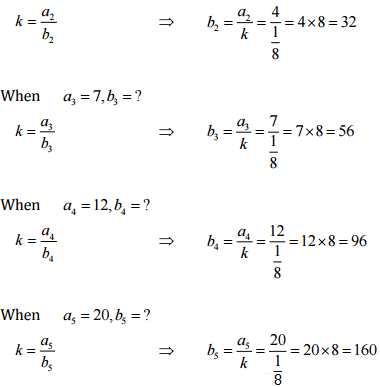Parts of red pigment 1 4 7 12 20 Parts of base 8 32 56 96 160

Question 3:

In Question 2 above, if 1 part of a red pigment requires 75 mL of base, how much red pigment should we mix with 1800 mL of base?

Let the parts of red pigment mix with 1800 mL base be x

 Parts of red pigment 1 X Parts of base 75 1800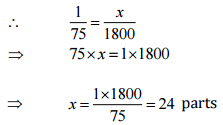Hence, with base 1800 mL, 24 parts red pigment should be mixed.

Question 4:

A machine in a soft drink factory fills 840 bottles in six hours. How many bottles will it fill in five hours?

Let the number of bottles filled in five hours be x.

 Hours 1 X Bottles 75 1800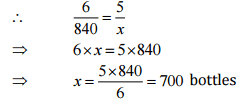Hence, the number of bottles filled in five hours be 700.

Question 5:

A photograph of a bacteria enlarged 50,000 times attains a length of 5 cm as shown In the diagram. What is the actual length of the bacteria? If the photograph is enlarged 20,000 times only, what would be its enlarged length?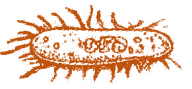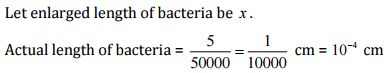Length 5 X Enlarged length 50,000 20,000

Here length and enlarged length of bacteria are in direct proportion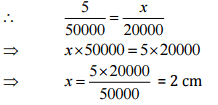Question 6:

In a model of a ship, the mast is 9 cm high, while the mast of the actual ship is 12 m high. If the length if the ship is 28 m, how long is the model ship?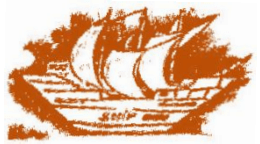Let the length of model ship be x

 Length of actual ship (in m) 12 28 Length of model ship (in cm) 9 X

Here length of mast and actual length of ship are in direct proportion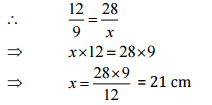Hence, the length of the model ship is 21 cm.

Question 7:

Suppose 2 kg of sugar contains 9 x 106 crystals. How many sugar crystals are there in

(i) 5 kg of sugar?

(ii) 1.2 kg of sugar?

Let sugar crystals be x.

 Weight of sugar (in kg) 2 5 No. of crystals 9x106 X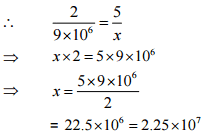Hence, the number of sugar crystals is 2.25 x 107.

(ii)

Let sugar crystals be x.

 Weight of sugar (in kg) 2 1.2 No. of crystals 9x106 X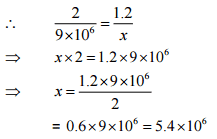Hence, the number of sugar crystals is 5.4 x 106.

Question 8:

Rashmi has a road map with a scale of 1 cm representing 18 km. She drives on a road for 72 km. What would be her distance covered in the map?

Let distance covered in the map be x.

 Actual distance (in km) 18 72 Distance covered in map (in cm) 1 X

Here actual distance and distance covered in the map are in direct proportion.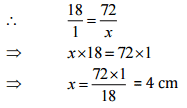Hence, distance covered in the map is 4 cm

Question 9:

A 5 m 60 cm high vertical pole casts a shadow 3 m 20 cm long. Find at the same time

(i) the length of the shadow cast by another pole 10 m 50 cm high

(ii) the height of a pole which casts a shadow 5 m long.

Here height of the pole and length of the shadow are in direct proportion.

And 1 m = 100 cm

5 m 60 cm = 5 x 100 + 60 = 560 cm

3 m 20 cm = 3 x 100 + 20 = 320 cm

10 m 50 cm = 10 x 100 + 50 = 1050 cm

5 m = 5 x 100 - 500 cm

(i) Let the length of the shadow of another pole be x.

 Height of pole (in cm) 560 1050 Length of shadow (in cm) 320 X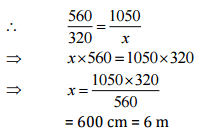Hence, the length of the shadow of another pole be 6m.

Let the hight of the pole be x.

 Height of pole (in cm) 560 x Length of shadow (in cm) 320 500Hence, the hight of the pole be 8m 75 cm

Question 10:

A loaded truck travels 14 km in 25 minutes. If the speed remains the same, how far can it travel in 5 hours?

Let distance covered in 5 hours be .r km.

1 hour = 60 minutes

5 hours = 5 x 60 = 300 minutes

 Distance (in km] 14 X Time (in minutes) 25 300

Here distance covered and time in direct proportion.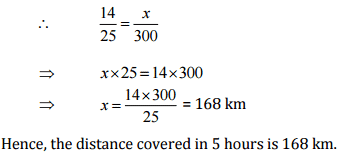The document NCERT Solutions (Ex - 13.1) - Direct and Inverse Proportions Notes - Class 8 is a part of Class 8 category.
All you need of Class 8 at this link: Class 8
 Use Code STAYHOME200 and get INR 200 additional OFF

Track your progress, build streaks, highlight & save important lessons and more!

,

,

,

,

,

,

,

,

,

,

,

,

,

,

,

,

,

,

,

,

,

;